# Chain space

(diff) ← Older revision | Latest revision (diff) | Newer revision → (diff)

An incidence system to axiomatically describe chain geometries (cf. Chain geometry).

A weak chain space is an incidence system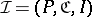satisfying the three conditions below. Here, the elements ofare called chains and two different points (i.e., elements of) are called distant if they are incident with a common chain.

i) any three pairwise distant points are contained in exactly one chain;

ii) any chain contains at least three points;

iii) any point lies in at least one chain.

For a point, let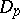be the set of all points distant toand let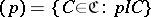. Then the incidence system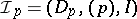is called the residual space of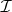at.

A partial parallel structureis an incidence system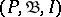together with an equivalence relationon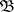satisfying the two conditions below. Here, the elements ofare called lines.

a) two different points are incident with at most one line;

b) for a lineand point, there is exactly one line,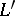, incident withand such that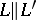. Condition b) is the Euclid parallel axiom.

A partial parallel structure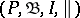is called a partial affine space if there is an affine spacesuch thatis the set of points of,is the set of straight lines ofandis the natural parallelism on.

A weak chain space is called a chain space if all residual spaces of it are partial affine spaces.

Every proper chain geometry is a chain space. Conversely, the chain spaces that are proper chain geometries can be characterized by suitable automorphism groups [a1].

A contact space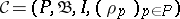is a weak chain spacetogether with a family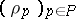, where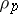is an equivalence relation on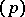with the following properties:

1) if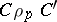, thenis the only point common toand;

2) if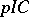andis a point distant to, then there is a unique chain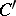incident withandfor which.

Clearly, for a contact spaceany residual space of the incidence systemgives rise to a partial parallel structure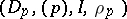. Conversely, any chain spaceis a contact space (taking forthe natural parallelism of the affine space underlying). One can characterize the contact spaces that are chain spaces by certain configurations together with richness conditions [a3].

An affine chain space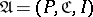is a contact space, whereis the point set of an affine space. The elements of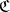are called affine chains and are normal rational curves in, i.e., affine parts of curves which are a Veronese variety (cf. Veronese mapping). For the setof all affine chains that are straight lines, the structure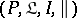is a partial affine space. Affine chain spaces can be constructed by means of Jordan algebras [a2] (cf. also Jordan algebra).

A classical example is the quadric model of a chain space, constructed on a quadricby means of plane sections. Moreover, the stereographic projection from a simple pointof(to a hyperplane different from the tangent plane ofat) then gives rise to an affine chain space (cf. also Benz plane).

How to Cite This Entry:
Chain space. Encyclopedia of Mathematics. URL: http://encyclopediaofmath.org/index.php?title=Chain_space&oldid=12810
This article was adapted from an original article by A. Herzer (originator), which appeared in Encyclopedia of Mathematics - ISBN 1402006098. See original article